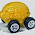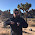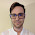## Friday, May 22, 2015

### The rest of the Solow model

Here, I mostly referred to the Cobb-Douglas production function piece, not the piece of the Solow model responsible for creating the equilibrium level of capital. That part is relatively straight-forward. Here we go ...

Let's assume two additional information equilibrium relationships with capital $K$ being the information source and investment $I$ and depreciation $D$ (include population growth in here if you'd like) being information destinations. In the notation I've been using: $K \rightarrow I$ and $K \rightarrow D$.

This immediately leads to the solutions of the differential equations:

$$\frac{K}{K_{0}} = \left( \frac{D}{D_{0}}\right)^{\delta}$$

$$\frac{K}{K_{0}} = \left( \frac{I}{I_{0}}\right)^{\sigma}$$

Therefore we have (the first relationship coming from the Cobb-Douglas production function)

$$Y \sim K^{\alpha} \text{ , }\;\;\;\; I \sim K^{1/\sigma} \text{ and }\;\;\;\; D \sim K^{1/\delta}$$

If $\sigma = 1/\alpha$ and $\delta = 1$ we recover the original Solow model, but in general $\sigma > \delta$ allows there to be an equilibrium. Here is a generic plot:

Assuming the relationships $K \rightarrow I$ and $K \rightarrow D$ hold simultaneously gives us the equilibrium value of $K = K^{*}$:

$$K^{*} = K_{0} \exp \left( \frac{\sigma \delta \log I_{0}/D_{0}}{\sigma - \delta} \right)$$

As a side note, I left the small $K$ region off on purpose. The information equilibrium model is not valid for small values of $K$ (or any variable). That allows one to choose parameters for investment and depreciation that could be e.g. greater than output for small $K$ -- a nonsense result in the Solow model, but just an invalid region of the model in the information equilibrium framework.

An interesting add-on is that $Y$ and $I$ have a supply and demand relationship in partial equilibrium with capital being demand and investment being supply (since $Y \rightarrow K$, by transitivity they are in information equilibrium). If $s$ is the savings rate (the price in the market $Y \rightarrow I = Y \rightarrow K \rightarrow I$), we should be able to work out how it changes depending on shocks to demand. There should be a direct connection to the IS-LM model as well.

1.Wow you have been on this growth theory stuff for a bit now, now that you are pretty much an expert you should dig into piketty and his critics. There are some fundamental disagreements about the definition of capital that lead to strikingly different results.

1.Also I'm not sure if you are aware, but perusing say the old model or model, etc might help you gain some perspective on the ideological line up behind assumptions like decreasing/constant returns to scale etc. It is more informative I think to follow the history of political thought in universities and line it up with models to understand the development of growth theory. That is how I teach it to students anyway. You can also take a stab at the Soviet and India models of growth theory. I think most of this is wiki knowledge...

2.Old kaldor model*

3.Hi, LAL.

Just returned from vacation and so responses to your comments will probably trickle out over time.

To tell the truth, I'm not sure I understand the full Piketty picture of the future path of economic growth and the capital share. I remember reading about some issues with how he treated depreciation that got some people in a tizzy. That seems like a small issue given that not only does productivity dominate the observed data, but the growth models don't describe the observed data very well.

Additionally, "balanced growth" at some constant rate g for an indefinite period of time seems to be impossible in the information equilibrium picture.

Regarding the definitions of capital, my reponse is and exasperated: "I know, right?"

Growth is capital and labor, but we don't have a universally accepted definition of capital, and we still need the total factor productivity fudge factor to make it come out ... I mean, whaaa? Maybe the definition of capital should be whatever makes TFP constant ...

The law of large numbers might save you again here ... what ever collection of things added together should end up being proportional to the Platonic ideal of capital. At least I hope.

4.Also -- I'd look at any model; I'll check out Kaldor ...

2.Sorry, just started a new job so am reading a month's worth of posts...assuming real variables in the Solow model is meant to paint the moral picture first. It is agnostic to the mechanism, planner vs market...which is part of its appeal. Obviously taking it too literally without inflation has problems...but to me it is something like developing special relativity vs General relativity. Special came first in my opinion be cause it was easier and answered the questions and posed the riddles it needed to....I think the history of growth theory had been about the same...

1.I admit we have no General theory no matter how loudly krugman trumpets The General Theory

2.I was under the impression that the economic growth models are built in terms of real variables because the idea of real vs nominal growth had been embedded in economics since Hume. If some part of the rise in the price of everything was due simply to money, it makes perfect sense to want to call the remaining bit "real" growth and therefore "adjust" for inflation.

I have no problem with that or with Solow as a step in the development of better models -- I think that is the consensus among economists. Romer in his critique looked to the Solow model as the last common ancestor for "scientific" and "mathy" models.

Additionally, I looked more closely at the structure of the Solow model in the information equilibrium framework -- it is supposed to have a scale invariance, but the only way to keep it is to have time dependent "parameters" ... i.e. if $K \rightarrow a(t) K$ and $Y \rightarrow a(t) Y$, then you should take $K_{0} \rightarrow a(t) K_{0}$ and $Y_{0} \rightarrow a(t) Y_{0}$ and you get:

$$\frac{a(t) Y}{a(t) Y_{0}} = \left( \frac{a(t) K}{a(t) K_{0}} \right)^{\alpha} \left( \frac{L}{L_{0}} \right)^{\beta}$$

Krugman seems to look at Keynes as the last common ancestor of "good" and "bad" economics -- I'd actually say Hicks and the diagrammatic approaches, but that's a bias on my part because the information equilibrium view says the diagrammatic approaches should work pretty well ...

3.This comment has been removed by the author.

4.Just to preempt a lecture..im not saying Solow growth theory is as well founded as special relativity...I'm saying it appropriately addresses the intellectual climate of time in a manner similar to I think Einstein did when he wrote his early papers on relativity or say on Brownian motion?... My memory is fuzzy there...

1.Assuming the Solow model turns out to be a good model in the final theory of everything in economics, then I'd probably use Einstein's work on the photoelectric effect here since that paper explained something that was baffling (because it was quantum). It was on the path to eventual understanding, but was still 20+ years before QM was developed. Relativity was a paper that cleared out bad models (the aether) -- it represented "new physics" and a great leap in understanding. Brownian motion was a paper that was 'clever' and useful -- chemists could use it to accurately measure Avogadro's number. It was an application of existing physics. Given those descriptions in terms of (my opinion) of physicists views, which one sounds best to you?

5.This comment has been removed by the author.

6.Somewhat unrelated, but how would entropy maximising versions of neoclassical firms and agents work to determine labour/leisure choice? ( basically, can you expand the ITM solow model to include wages and endogenous labour supply growth?)

1.I've looked at the model p : N → NW where NW is nominal wages; the price in that model is approximately constant so that nominal output N ≈ 2.1 NW ... i.e. p = 2.1 and the IT index for the model is 1.

http://informationtransfereconomics.blogspot.com/2014/02/sticky-wages-information-transfer-and.html

However, the statement "p ≈ 2.1" and the statement that the "employment population ratio is approximately constant" have the same fidelity in the information transfer model. That is to say deviations from wages being a constant fraction of output and deviations from the employment-population ratio being constant are both on the order of the error of the model.

Although it is frequently shown zoomed in so that its fluctuations cover the entire y-axis, the employment-population ratio is remarkably constant for an economic indicator. It has about the same spread as the unemployment rate -- another case where the information transfer model essentially says it's constant (on average, over a long period, etc).

Basically, in this model, adding those pieces ends up being fairly trivial, but they don't add much information.

Comments are welcome. Please see the Moderation and comment policy.

Also, try to avoid the use of dollar signs as they interfere with my setup of mathjax. I left it set up that way because I think this is funny for an economics blog. You can use € or £ instead.